# GSEB Solutions Class 6 Maths Chapter 1 Knowing Our Numbers Ex 1.3

Gujarat Board GSEB Textbook Solutions Class 6 Maths Chapter 1 Knowing Our Numbers Ex 1.3 Textbook Questions and Answers.

## Gujarat Board Textbook Solutions Class 6 Maths Chapter 1 Knowing Our Numbers Ex 1.3

Question 1.
Estinwie each of the following using general rule:
(a) 730 + 998
(b) 796 – 314
(c) 12.904 + 2,888
(d) 28,292 – 21.496
Make ten more such examples of addition and subtraction, and estimate their outcome.
Solution:
(a) 730 + 998
730 → 700 [Rounded off to hundreds]
998 → 1000 [Rounded off to hundreds]
Estimated sum = 700 + 1000 = 1700

(b) 796 – 314
796 → 800 [Rounded off to hundreds]
314 → 300 [Rounded off to hundreds]
Estimated difference 800 – 300 = 500

(c) 12,904 + 2888
12,904 → 13,000 [Rounded off to hundreds]
2,888 → 3,000 [Rounded off to hundreds]
Estimated sum = 13,000 + 3,000 = 16,000

(d) 28,292 – 21,496
28,292 → 28,000 [Rounded off to hundreds]
21,496 → 21,000 [Rounded off to hundreds]
Estimated difference = 28000 – 21000 = 7000
Note: Make ten more such examples and do yourself.Question 2.
Give a rough estimate (By rounding off to nearest hundreds) and also a closer estimate (by rounding off to nearest tens):
(a) 439 + 334 + 4,317
(b) 1,08,734 – 47,599
(c) 8,325 – 491
(d) 4,89,348 – 48,365
Solution:
(a) 439 + 334 + 4,317Rough estimate 400 + 300 + 4300 = 5,000 Again,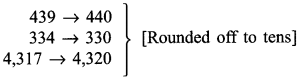Closer estimate = 440 + 330 + 4,320 = 5,090

(b) 1,08,734 – 47,599
Since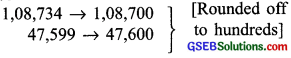Rough estimate = 1,08,700 – 47,600 = 61,100 Again,Closer estimate = 1,08,730 – 47,600 = 61,130

(c) 8,325 – 491
Since,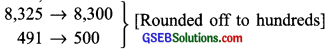Rough estimate 8,300 – 500 = 7,800 Again.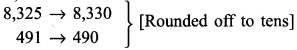Closer estimate = 8,330 – 490 = 7,840

(d) 4,89,348 – 48,365
Since,Rough estimate = 4,89,300 – 48,400 = 4,40,900 Again,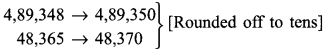Closer estimate = 4,89,350 – 48,370 = 4,40,980
Note: Make four more such examples and do yourself.Question 3.
Estimate the following products using general rule:
(a) 578 x 161
(b) 5,281 x 3,491
(c) 1,291 x 592
(d) 9,250 x 29
Make four more such examples and do yourself.
Note: Round the numbers up or down before the calculation.
Solution:
(a) 578 x 161
578 → 600 [Rounding off to hundreds]
161 → 200 [Rounding off to hundreds]
∴Estimated product 600 x 200 = 1,20,000

(b) 5,281 x 3,491
5,281 → 5,000 [Rounding off to thousands]
3,491 → 3,500 [Rounding off to hundreds]
∴Estimated product = 5,000 x 3,500 = 1,75,00,000

(c) 1,291 x 592
1,291 → 4 1,300 [Rounding off to hundreds]
592 → 600 [Rounding off to hundreds]
∴Estimated product = 1,300 x 600 = 7,80,000

(d) 9250 x 29
9,250 → 9,300 [Rounding off to hundreds]
29 → 30 [Rounding off to tens]
∴Estimated product = 9300 x 30 = 279000
Note: Make four more such examples and do yourself.